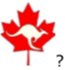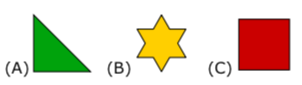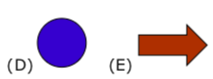1. Part A: Each correct answer is worth 3 points

Ann has seven cards with letters as shown in the picture.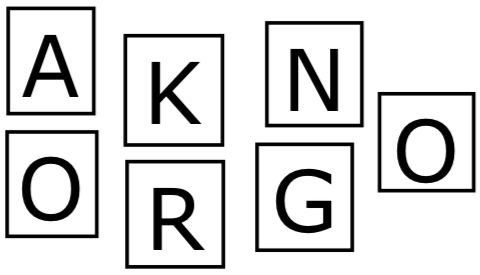Which other card does she need in order to make the word KANGAROO?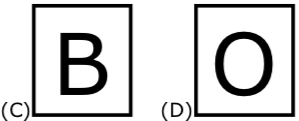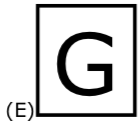2. In Ryan’s yard rabbits and squirrels left tracks in the snow.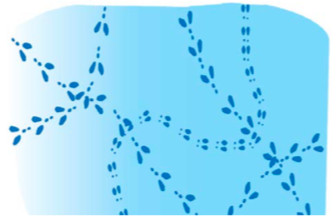How many animals were there if each animal only walked through once?

3. How many triangles are there in the picture?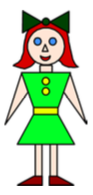4. Find the missing part of the house.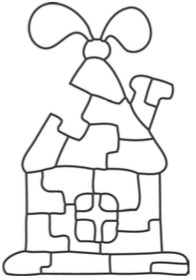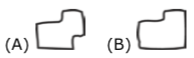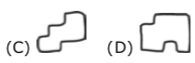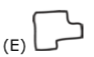5. Five ladybugs live on a bush. How many spots, in total, do the ladybugs have?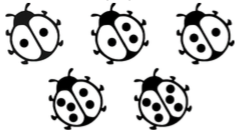6. Eric had ten equal metal strips.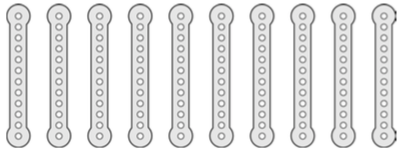He screwed pairs of them together into five long strips as shown below.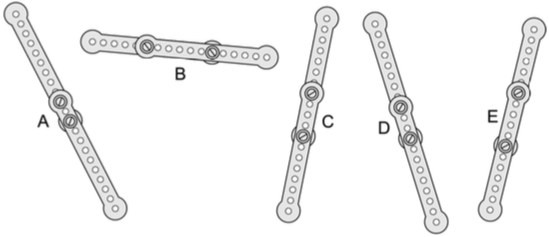Which strip is the shortest?

7. Part B: Each correct answer is worth 4 points

Which of the scarecrows has a pointy hat, a checkered shirt, and three patches on its pants?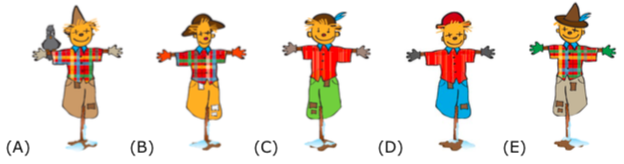8. Marko has 9 candies and Tomo has 17 candies. How many candies does Tomo need to give to Marko so that each boy will have the same number of candies?

9. Don made two bricks by sticking cubes together as shown below.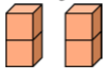Which structure could not be built using the two bricks?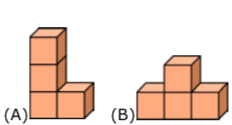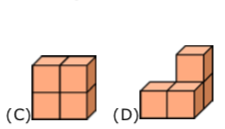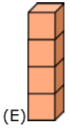10. Six towers were built with grey cubes and white cubes. Each tower was made with five cubes. Cubes of the same colors do not touch. How many white cubes are there?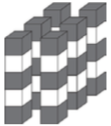11. Kanga can jump from one circle to the next only if they are connected by a line. She cannot jump into any circle more than once. Kanga starts from circle S and makes exactly 4 jumps to get to circle F. In how many different ways can Kanga do this?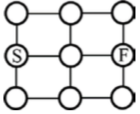12. What is the minimum number of shapes that must be removed so that only shapes of one type will remain?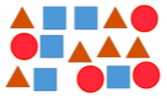13.

Put the numbers 1, 2, 3, 4, 5 in the boxes so that everything is correct.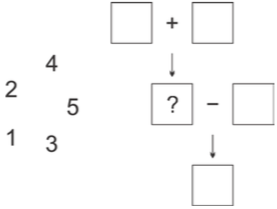Which number goes into the box marked with the question mark?

14. A ship was attacked by pirates. One by one they climbed a long rope ladder to get to the ship. The pirate captain was in the middle. If the captain was the eighth pirate to get on the ladder, then how many pirates were there on the ladder?

15. The numbers 3, 5, 7, 8 and 9 were written into the squares so that the sum of the numbers in the row is equal to the sum of the numbers in the column. Which number was written in the centre square?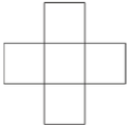16. Basil attached five stickers with the digits 1, 2, 3, 4, 5 in some order on a sheet of paper as shown in the picture.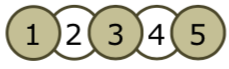In what order could Basil have put the stickers?

17. Nelly took two square cards and wrote the four digits 2, 0, 1, 5, as shown.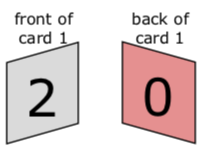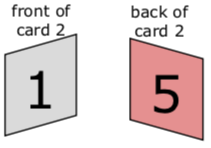In how many ways can Nelly make two-digit numbers using her cards?

18. The following six shapes were drawn on the six walls of a cube: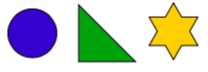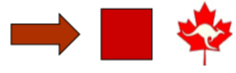Below is what you see if looking at this cube from two positions.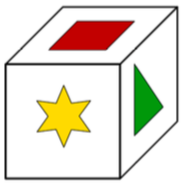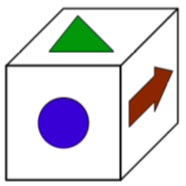Which shape is opposite to the Canadian Math Kangaroo logo